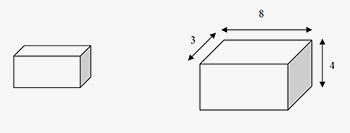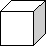# 3 D geometry- Mensuration-Cube & Cuboid

###### Understanding Three-Dimensional Geometry:
Most tests often include questions based on the knowledge of the geometries of 3-D objects such as cylinder, cone, cuboid, cube & sphere. The purpose of the article is to help you learn basics of 3-D geometry and encapsulate some of the important formulae and tricks.
The questions on Volume and Surface Area appear in all the competitive exams. Most of the students tend to avoid this topic considering it to be quite complex and calculative. This article would help you not only in memorizing the formulas, but also in understanding direct or indirect applications of these formulas. We strongly advice you go through each and every definition and formula given below to solve questions on Surface Area and Volume.
Solids: Solids are three–dimensional objects, bound by one or more surfaces. Plane surfaces of a solid are called its faces. The lines of intersection of adjacent faces are called edges.
For any regular solid, Number of faces + Number of vertices = Number of edges + 2. This formula is called Euler’s formula.
Volume: Volume of a solid figure is the amount of space enclosed by its bounding surfaces. Volume is measured in cubic units.
###### Surface area and Volume Formulas
Cuboid: A cuboid is a three-dimensional figure formed by six rectangular surfaces, as shown below.  Each rectangular surface is a face.  Each solid line segment is edge, and each point at which the edges meet is vertex. A rectangular solid has six faces, twelve edges, and eight vertices. Edges mean sides and vertices mean corners. Opposite faces are parallel rectangles that have same dimensions.
The surface area of a rectangular solid is equal to the sum of the areas of all the faces.
Total surface area = 2(lb + bh + hl )
Curved surface area = 2h(l + b)
Volume = lbh, Where l= length, b= breadth, h= height
In the rectangular solid, the dimensions are 3, 4, and 8.
The surface area of the rectangular solid is equal to 2[(3 × 4) + (3 × 8) + (4 × 8)] = 136.
The cuboid volume is equal to 3 × 4 × 8 = 96.
Body diagonal of a cuboid = Length of the longest rod that can be kept inside a rectangular room is =
√L2 + B2 + H21. Two cubes, each of edge 10 cm, are joined to form single cuboid. What is the surface area of the new cuboid so formed?
1. 1200 cm2
2. 1000 cm2
3. 1100 cm2
4. 600 cm2
Explanation: Here length of cuboid = 20 cm.
Breadth and height of cuboid = 10 cm.
∴TSA = 2(L*B + B*H + H*L)
surface area = 2(200 + 100 + 200) = 1000 cm2
Cube: A rectangular solid in which all the edges are equal is a cube. In a cube, just like cuboids, there are six faces, eight vertices & twelve edges.
cube Volume = a3.Surface Area formula = 6a2, where a is the side of a cube.
Body Diagonal = Length of the longest rod inside a cubical room = a√3
1. A cube of 1.7 litres volume will have each edge closest to
1. 18 cm
2. 24 cm
3. 12 cm
4. 36 cm
Explanation: Volume = 1.7 litres = 1.7 x 1000 = 1700 cm3.
∴Edge = (1700)1/3 = 12 cm.
2. The surface area of a cube is 486 cm2 and melted into small cubes, each of 54 mm2 surface area. Find the number of small cube
1. 27,000
2. 400
3. 8,000
4. 800
Explanation: Surface area of larger cube = 486cm2
∴6L2 = 486 ⇒ L2 = 81 ⇒ L = 9cm.
∴ Volume of larger cube = 9 x 9 x 9 = 729 cm3. Surface area of smaller cube = 54mm2 = 54/100 cm2. ∴6l2 = 54/100 ⇒ l2 = 0.09 ⇒ 1 = 0.3cm.
Volume of smaller cube = 0.3 x 0.3 x 0.3
= 0.027 cm3.
&there; x = 729/0.027 = 27000.